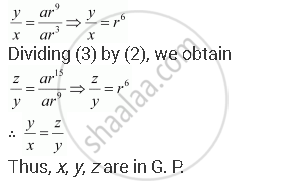Share

# If the 4th, 10th and 16th Terms of a G.P. Are X, Y and Z, Respectively. Prove that X, Y, Z Are in Geometric Progression - Mathematics

#### Question

If the 4th, 10th and 16th terms of a G.P. are x, y and z, respectively. Prove that x, y, z are in G.P.

#### Solution

Let a be the first term and r be the common ratio of the G.P.

According to the given condition,

a4 = a r3 = x … (1)

a10 = a r9 = y … (2)

a16 = a r15 z … (3)

Dividing (2) by (1), we obtainIs there an error in this question or solution?